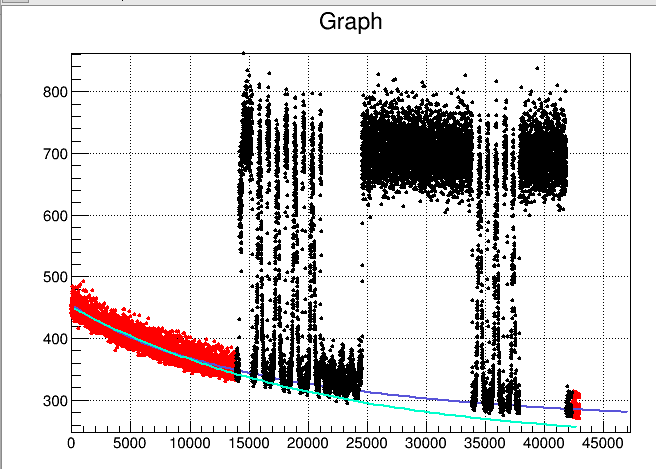# Unbinned chi2 fit for response model with RooFit

I have a dataset with (x, y) and I would like to fit it as chi2 but without passing through an histogram.

I would indeed like to minimize S=|y-f(x)|^2. Is there a way to describe this in RooFit?

Thanks, Guido

Hi,

about unbinned fits and chi2, I would recommend to read this thread: Error with Chi^2 fitting to unbinned dataset

If you want to perform an unbinned likelihood fit, the procedure is straightforward:

``````   RooRealVar x("x","x",0,10) ;

RooRealVar mean("mean","mean of gaussians",5) ;
RooRealVar sigma1("sigma1","width of gaussians",0.5) ;
RooRealVar sigma2("sigma2","width of gaussians",1) ;
RooGaussian sig1("sig1","Signal component 1",x,mean,sigma1) ;
RooGaussian sig2("sig2","Signal component 2",x,mean,sigma2) ;

RooRealVar a0("a0","a0",0.5,0.,1.) ;
RooRealVar a1("a1","a1",0.2,0.,1.) ;
RooChebychev bkg("bkg","Background",x,RooArgSet(a0,a1)) ;

RooRealVar sig1frac("sig1frac","fraction of component 1 in signal",0.8,0.,1.) ;

RooRealVar bkgfrac("bkgfrac","fraction of background",0.5,0.,1.) ;

auto d = model.generate(x,10000) ;

model.fitTo(*d) ;
``````

Cheers,
D

Thanks for the reply, I looked at the example and it is not obvious to me how do I apply it. You proposal looks to me performing a likelihood fit of the model, but this is not what i need.

As already pointed I have a 2-dimensional dataset, where each element is:

• time, measure

The model you propose is 1-d and the would just generate more data where the model has a larger response.

I will try but I doubt your proposal is correct.

My proposal illustrates how to perform an unbinned fit. The model is generated from scratch as an example. The 2-dimensional fit is analogous. The assumption of performing a chi2 unbinned fit debatable.

Hi,

Why do you need to use RooFit in this case. Your f(x) does not need to be a pdf, it is just a normal function and you don’t need to normalise.
Just put your points in a TGraph and call TGraph::Fit.

In case f(x) is described in RooFit as a RooAbsReal object, you can transform to a TF1 by calling the RooAbsReal::asTF function

Lorenzo

Hi Lorenzo, you are correct. I am trying to use RooFit because the next step would be to make a combined fit of multiple TGraph objects. Looks to me that this second part is easier to do in RooFit.

Guido

For your information, inspired by the previous comment the code that worked for me is as follow:

``````  RooRealVar ADCsd("ADCsd","ADC err",5,1,50);
RooRealVar rate1("rate1", "rate1 [Hz]",-1e-5,-1, -1e-7);
RooExponential ExpShape("ExpShape", "ExpShape", timeVar, rate1);
RooRealVar ped("ped","ped",200,100,500);
RooRealVar a1("a1","a1",100,0,300);
RooFormulaVar PedShape("PedShape","ped+a1*exp(rate1*eltime)",RooArgSet(ped, a1, rate1, timeVar));
``````

I have however to admit that it seems that I failed with this model: the Teal line is the RooFit Result while the violet one is the standard TGraph::Fit result.Hi,

If you have multiple Graphs you can use TMultiGraph for fitting,
However this works if the function is the same for all the Graphs.

Lorenzo

Thanks for the suggestion, I will look into this. However there will be some parameters that won’t be the same for the different graphs.

I am also still wondering what is wrong in this PDF.

Guido

Hi,

I would need your full running code to understand what is wrong with your PDF

Lorenzo

This topic was automatically closed 14 days after the last reply. New replies are no longer allowed.